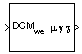# Direction Cosine Matrix to Wind Angles

Convert direction cosine matrix to wind angles

• Library:
• Aerospace Blockset / Utilities / Axes Transformations

•## Description

The Direction Cosine Matrix to Wind Angles block converts a 3-by-3 direction cosine matrix (DCM) into three wind rotation angles. The DCM matrix performs the coordinate transformation of a vector in earth axes (ox0, oy0, oz0) into a vector in wind axes (ox3, oy3, oz3). For more information on the direction cosine matrix, see Algorithms.

This implementation generates a flight path angle that lies between ±90 degrees, and bank and heading angles that lie between ±180 degrees.

## Ports

### Input

expand all

Direction cosine matrix, specified as a 3-by-3 matrix, to transform Earth-fixed vectors to wind-fixed vectors.

Data Types: `double`

### Output

expand all

Wind angles (bank, flight path, heading), returned as a 3-by-1 vector, in radians.

Data Types: `double`

## Parameters

expand all

Block behavior when the direction cosine matrix is invalid (not orthogonal).

• `Warning` — Displays warning indicating that the direction cosine matrix is invalid.

• `Error` — Displays error indicating that the direction cosine matrix is invalid.

• `None` — Does not display warning or error (default).

#### Programmatic Use

 Block Parameter: `action` Type: character vector Values: `'None'` | `'Warning'` | `'Error'` Default: `'None'`

Data Types: `char` | `string`

Tolerance of the direction cosine matrix validity, specified as a scalar. The block considers the direction cosine matrix valid if these conditions are true:

• The transpose of the direction cosine matrix times itself equals `1` within the specified tolerance (`transpose(n)*n == 1±tolerance`).

• The determinant of the direction cosine matrix equals `1` within the specified tolerance (`det(n) == 1±tolerance`).

#### Programmatic Use

 Block Parameter: `tolerance` Type: character vector Values: `'eps(2)'` | scalar Default: `'eps(2)'`

Data Types: `double`

## Algorithms

The DCM matrix performs the coordinate transformation of a vector in earth axes (ox0, oy0, oz0) into a vector in wind axes (ox3, oy3, oz3). The order of the axis rotations required to bring this about is:

1. A rotation about oz0 through the heading angle (χ) to axes (ox1, oy1, oz1)

2. A rotation about oy1 through the flight path angle (γ) to axes (ox2, oy2, oz2)

3. A rotation about ox2 through the bank angle (μ) to axes (ox3, oy3, oz3)

`$\begin{array}{l}\left[\begin{array}{c}o{x}_{3}\\ o{y}_{3}\\ o{z}_{3}\end{array}\right]=DC{M}_{we}\left[\begin{array}{c}o{x}_{0}\\ o{y}_{0}\\ o{z}_{0}\end{array}\right]\\ \\ \left[\begin{array}{c}o{x}_{3}\\ o{y}_{3}\\ o{z}_{3}\end{array}\right]=\left[\begin{array}{ccc}1& 0& 0\\ 0& \mathrm{cos}\mu & \mathrm{sin}\mu \\ 0& -\mathrm{sin}\mu & \mathrm{cos}\mu \end{array}\right]\left[\begin{array}{ccc}\mathrm{cos}\gamma & 0& -\mathrm{sin}\gamma \\ 0& 1& 0\\ \mathrm{sin}\gamma & 0& \mathrm{cos}\gamma \end{array}\right]\left[\begin{array}{ccc}\mathrm{cos}\chi & \mathrm{sin}\chi & 0\\ -\mathrm{sin}\chi & \mathrm{cos}\chi & 0\\ 0& 0& 1\end{array}\right]\left[\begin{array}{c}o{x}_{0}\\ o{y}_{0}\\ o{z}_{0}\end{array}\right]\end{array}$`

Combining the three axis transformation matrices defines the following DCM.

`$DC{M}_{we}=\left[\begin{array}{ccc}\mathrm{cos}\gamma \mathrm{cos}\chi & \mathrm{cos}\gamma \mathrm{sin}\chi & -\mathrm{sin}\gamma \\ \left(\mathrm{sin}\mu \mathrm{sin}\gamma \mathrm{cos}\chi -\mathrm{cos}\mu \mathrm{sin}\chi \right)& \left(\mathrm{sin}\mu \mathrm{sin}\gamma \mathrm{sin}\chi +\mathrm{cos}\mu \mathrm{cos}\chi \right)& \mathrm{sin}\mu \mathrm{cos}\gamma \\ \left(\mathrm{cos}\mu \mathrm{sin}\gamma \mathrm{cos}\chi +\mathrm{sin}\mu \mathrm{sin}\chi \right)& \left(\mathrm{cos}\mu \mathrm{sin}\gamma \mathrm{sin}\chi -\mathrm{sin}\mu \mathrm{cos}\chi \right)& \mathrm{cos}\mu \mathrm{cos}\gamma \end{array}\right]$`

To determine wind angles from the DCM, the following equations are used:

`$\begin{array}{l}\mu =\text{atan}\left(\frac{DCM\left(2,3\right)}{DCM\left(3,3\right)}\right)\\ \\ \gamma =\text{asin}\left(-DCM\left(1,3\right)\right)\\ \\ \chi =\text{atan}\left(\frac{DCM\left(1,2\right)}{DCM\left(1,1\right)}\right)\end{array}$`

## Version History

Introduced before R2006a